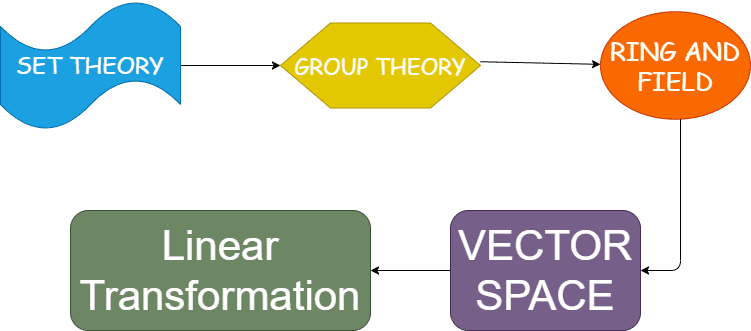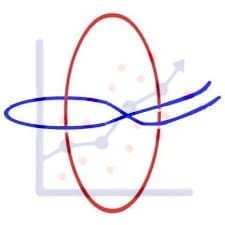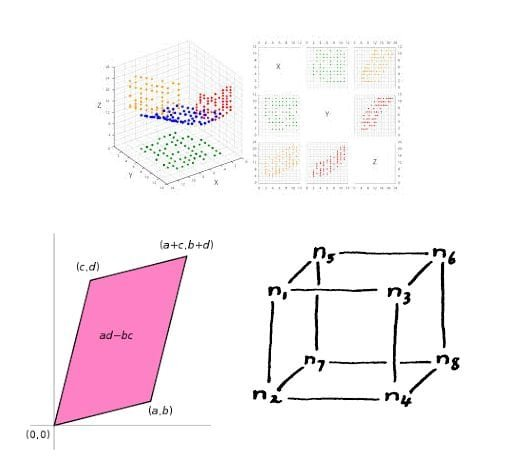Categories
College Mathematics

# Linear Transformation – IIT JAM 2018 Question Number 21

Try this beautiful problem from IIT JAM 2018. It involves the concept of vector spaces and linear mapping. We provide sequential hints so that you can try the problem.

## Competency in Focus: Linear Transformation

This problem from IIT JAM 2018 is based on calculation of linear transformation. It is Question no. 21 of the IIT JAM 2018 Problem series.

## First look at the knowledge graph:-## Next understand the problem

Let $U, V, \textbf{and}\quad W$ be finite dimensional real vector spaces, $T:U\rightarrow V, S: V\rightarrow W, P: W\rightarrow U$ be linear transformations. If range($ST$)=nullspace($P$), nullspace($ST$)=range ($P$) and rank($T$)=rank($S$), Then which one of the following is true? $\textbf{(A)}$ nullity of $T$ = nullity of $S$ $\textbf{(B)}$ dimension of $U \neq$  dimension of $W$ $\textbf{(C)}$ If dimension of $V = 3$, If dimension of $U = 4$, then $P$ is not identically zero. $\textbf{(C)}$ If dimension of $V = 4$, If dimension of $U = 3$, and $T$ is one-one then $P$ is identically zero.
##### Source of the problem
IIT JAM 2018, Question Number 21

### Linear transformation and Vector Space.

7/10
##### Suggested Book

Do you really need a hint? Try it first!
I want to make an opinion that this question is not hard but it is time consuming. So in a time paced exam beware of this kind of question. The fact I’ve used here : i) Ker$(ST)={0} \iff ST \quad \textbf{is injective}$ ii) $ST\quad \textbf{is injective} \iff$T$\quad \textbf{is injective}$ iii) And creating the counter examples which are  most time consuming. So, in hint 1, I want to disclose the answer and I can first try to find out the counter example by yourself. $\bullet \quad\textbf{The correct option is} \quad[\textbf{C}]$
Consider $U=V=W=\mathbb{R}^2$ and the maps areThe range($ST$) =$S_p\{c_1\}=$ Nullspace($P$). range($P$)=$S_p\{c_2\}=$ Nullspace($ST$) & rank($T$) $=1=$ rank ($S$) But, Nullity of $T=1 \neq 2=$ Nullity of $S$. And dim($U$) = dim($W$) = 2 So, the option $(A)$ and $(B)$ are incorrect.
Consider $U= \mathbb{R}^3,V= \mathbb{R}^4, W= \mathbb{R}^5$range ($ST$) = $S_p\{c_1,c_2\}=$ Nullspace($P$) Nullspace ($ST$)=$S_p\{c_3\}$=Range($P$) rank($T$)=$2$=rank($S$) and $P$ is not zero map So, option $(D)$ is not correct. Hence option $(C)$ is the one left which has to be true, Now lets prove that.
We’ll prove that $P$ is non zero. Suppose $P=0$ $U\longrightarrow V\longrightarrow W$ dim($U$) = $4$, dim($V$) =$3$  Now,         range ($P$)  $=0=$ Nullspace ($ST$)         $\Rightarrow ST$ is injective         $\Rightarrow T$ is injective         $\Rightarrow$ dim ($V\geq 4$)         Which is a contradiction. Hence $P=0$ And we are done

# College Mathematics Program

The higher mathematics program caters to advanced college and university students. It is useful for I.S.I. M.Math Entrance, GRE Math Subject Test, TIFR Ph.D. Entrance, I.I.T. JAM. The program is problem driven. We work with candidates who have a deep love for mathematics. This program is also useful for adults continuing who wish to rediscover the world of mathematics.## ISI MStat PSB 2006 Problem 8 | Bernoullian Beauty

This is a very simple and regular sample problem from ISI MStat PSB 2009 Problem 8. It It is based on testing the nature of the mean of Exponential distribution. Give it a Try it !## ISI MStat PSB 2009 Problem 8 | How big is the Mean?

This is a very simple and regular sample problem from ISI MStat PSB 2009 Problem 8. It It is based on testing the nature of the mean of Exponential distribution. Give it a Try it !## ISI MStat PSB 2009 Problem 4 | Polarized to Normal

This is a very beautiful sample problem from ISI MStat PSB 2009 Problem 4. It is based on the idea of Polar Transformations, but need a good deal of observation o realize that. Give it a Try it !## ISI MStat PSB 2009 Problem 6 | abNormal MLE of Normal

This is a very beautiful sample problem from ISI MStat PSB 2009 Problem 6. It is based on the idea of Restricted Maximum Likelihood Estimators, and Mean Squared Errors. Give it a Try it !## ISI MStat PSB 2009 Problem 3 | Gamma is not abNormal

This is a very simple but beautiful sample problem from ISI MStat PSB 2009 Problem 3. It is based on recognizing density function and then using CLT. Try it !## ISI MStat PSB 2009 Problem 1 | Nilpotent Matrices

This is a very simple sample problem from ISI MStat PSB 2009 Problem 1. It is based on basic properties of Nilpotent Matrices and Skew-symmetric Matrices. Try it !## ISI MStat PSB 2006 Problem 2 | Cauchy & Schwarz come to rescue

This is a very subtle sample problem from ISI MStat PSB 2006 Problem 2. After seeing this problem, one may think of using Lagrange Multipliers, but one can just find easier and beautiful way, if one is really keen to find one. Can you!

## Problem on Inequality | ISI – MSQMS – B, 2018 | Problem 2a

Try this problem from ISI MSQMS 2018 which involves the concept of Inequality. You can use the sequential hints provided to solve the problem.## Data, Determinant and Simplex

This problem is a beautiful problem connecting linear algebra, geometry and data. Go ahead and dwelve into the glorious connection.

## Problem on Integral Inequality | ISI – MSQMS – B, 2015

Try this problem from ISI MSQMS 2015 which involves the concept of Integral Inequality and real analysis. You can use the sequential hints provided to solve the problem.

This site uses Akismet to reduce spam. Learn how your comment data is processed.